# C++ program to print the multiplication table of a number

In this article, you will learn and get code to print a multiplication table of numbers using a C++ program. Here is the list of programs for printing the multiplication table:

### What is a multiplication table?

A multiplication table shows a list of multiples of a particular number, from 1 to 10. For instance, the multiplication table for 3 is:

```3 * 1 = 3
3 * 2 = 6
3 * 3 = 9
3 * 4 = 12
3 * 5 = 15
3 * 6 = 18
3 * 7 = 21
3 * 8 = 24
3 * 9 = 27
3 * 10 = 30```

Note: Typically, the list of multiples is from 1 to 12. But in this article, we've created all the programs for printing the multiplication table based on multiples from 1 to 10 only.

## Print Multiplication Table of 2 in C++

```#include<iostream>
using namespace std;
int main()
{
int num=2, i, res;
for(i=1; i<=10; i++)
{
res = num*i;
cout<<num<<" * "<<i<<" = "<<res;
cout<<endl;
}
cout<<endl;
return 0;
}```

This program was built and runs under the Code::Blocks IDE. Here is its sample output: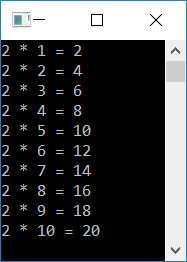The dry run of this program goes like this:

• Initially, num = 2
• Now the execution of the for loop begins. Its initialization (first statement) gets executed at first, but only once.
• So 1 gets initialized to i, and the condition i<=10 or 1<=10 evaluates to be true. Therefore, program flow goes inside the loop.
• And then num*i or 2*1 or 2 is set to res.
• Now, using the following statement:
`cout<<num<<" * "<<i<<" = "<<res;`
2 * 1 = 2, gets printed on the output.
• The third statement:
`cout<<endl;`
gets executed, which prints a newline.
• Now the program flow goes to the update (third statement) of the for loop and increments the value of i. So i=2
• The condition i<=10 or 2<=10 again evaluates to be true, therefore the program flow again goes inside the loop.
• This process continues until its condition is evaluated as false.
• In this way, the multiplication table of 2 gets printed on the output screen.

## In C++, print a multiplication table for any given number

To print a table of any given number in C++ programming, you have to ask the user to enter the number. Then start multiplying that number from 1 to 10, one by one, and display the multiplication result at the time of multiplying, as shown here in the following program.

```#include<iostream>
using namespace std;
int main()
{
int num, i, res;
cout<<"Enter the Number: ";
cin>>num;
for(i=1; i<=10; i++)
{
res = num*i;
cout<<num<<" * "<<i<<" = "<<res;
cout<<endl;
}
cout<<endl;
return 0;
}```

Here is the initial output produced by this C++ program: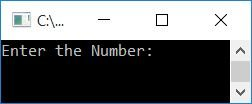Now supply the input, say 5, and press the ENTER key to print its table as shown in the snapshot given below: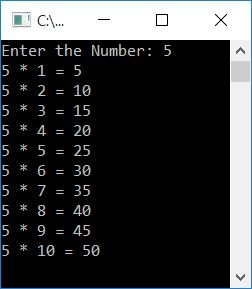## Print the multiplication table from 1 to 10 in C++

This program prints tables from 1 to 10. That is, first table 1 gets printed, then table 2 gets printed, and so on up to table 10:

```#include<iostream>
using namespace std;
int main()
{
int num, i, j, res;
cout<<"-----------Table from 1 to 10--------\n\n";
for(i=1; i<=10; i++)
{
num = i;
cout<<"Table of "<<num<<": ";
for(j=1; j<=10; j++)
{
res = num*j;
cout<<res<<"  ";
}
cout<<endl;
}
cout<<endl;
return 0;
}```

Here is the sample output produced by this program: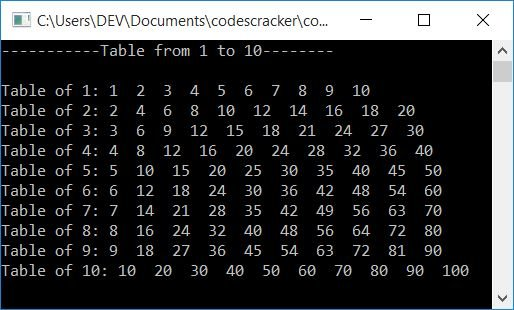## In C++, print a Multiplication Table within a Specific Range

This program allows the user to enter a range and print a table with all of the numbers in that range. For example, if the user enters a range of 3 and 15, then a table of all numbers from 3 to 15 gets printed.

```#include<iostream>
using namespace std;
int main()
{
int numOne, numTwo, num, i, j, res;
cout<<"Enter the Range: ";
cin>>numOne>>numTwo;
cout<<"--------Table from "<<numOne<<" to "<<numTwo<<"--------\n\n";
for(i=numOne; i<=numTwo; i++)
{
num = i;
cout<<"Table of "<<num<<": ";
for(j=1; j<=10; j++)
{
res = num*j;
cout<<res<<"  ";
}
cout<<endl;
}
cout<<endl;
return 0;
}```

Here's an example run with user input: 3 for the first number and 15 for the second: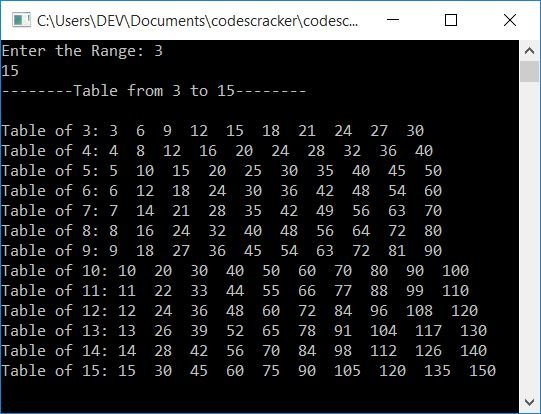What if the user enters two numbers in such a way that the first number is greater than the second?
To solve this problem, use this program instead of the previous one:

```#include<iostream>
using namespace std;
int main()
{
int numOne, numTwo, num, i, j, res;
cout<<"Enter the Range: ";
cin>>numOne>>numTwo;
if(numTwo>numOne)
{
cout<<"--------Table from "<<numOne<<" to "<<numTwo<<"--------\n\n";
for(i=numOne; i<=numTwo; i++)
{
num = i;
cout<<"Table of "<<num<<": ";
for(j=1; j<=10; j++)
{
res = num*j;
cout<<res<<"  ";
}
cout<<endl;
}
}
else
{
cout<<"--------Table from "<<numTwo<<" to "<<numOne<<"--------\n\n";
for(i=numTwo; i<=numOne; i++)
{
num = i;
cout<<"Table of "<<num<<": ";
for(j=1; j<=10; j++)
{
res = num*j;
cout<<res<<"  ";
}
cout<<endl;
}
}
cout<<endl;
return 0;
}```

Here is a sample run with user input, the first number being 20 and the second being 6: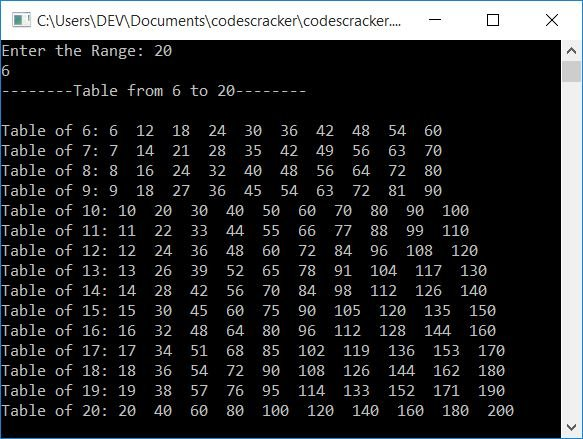#### The same program in different languages

C++ Quiz

« Previous Program Next Program »